Miscellaneous

Chapter 10 Class 11 Straight Lines
Serial order wise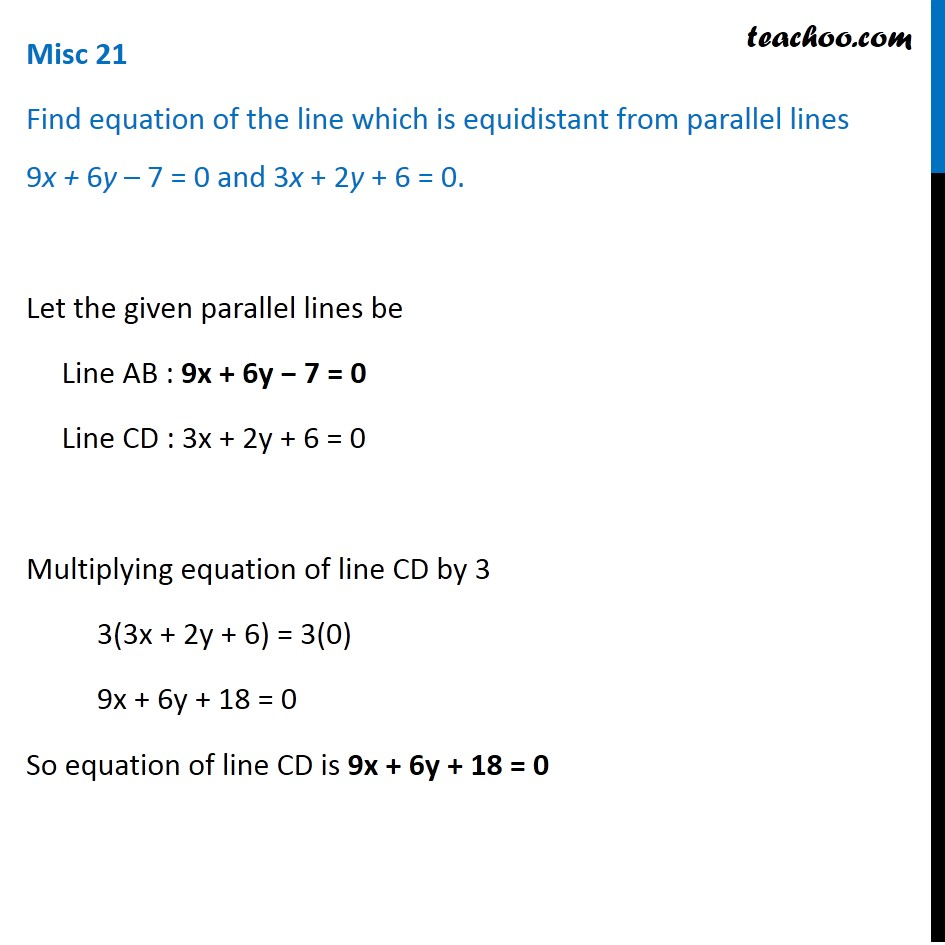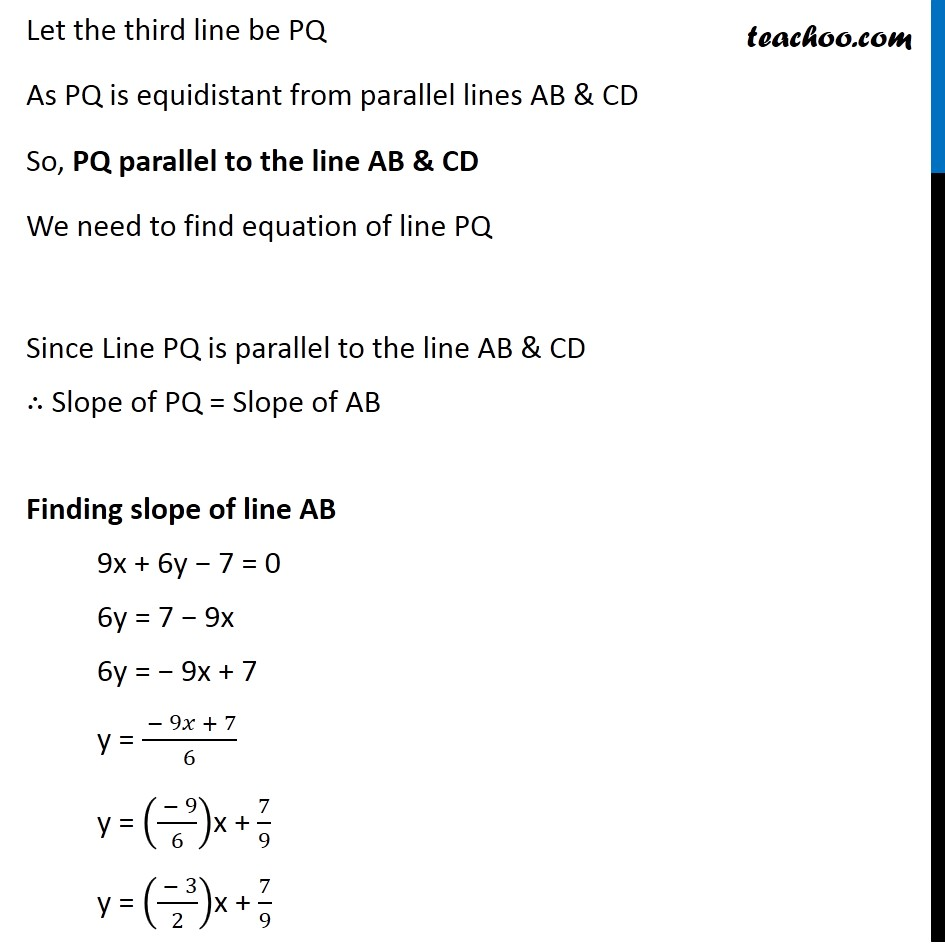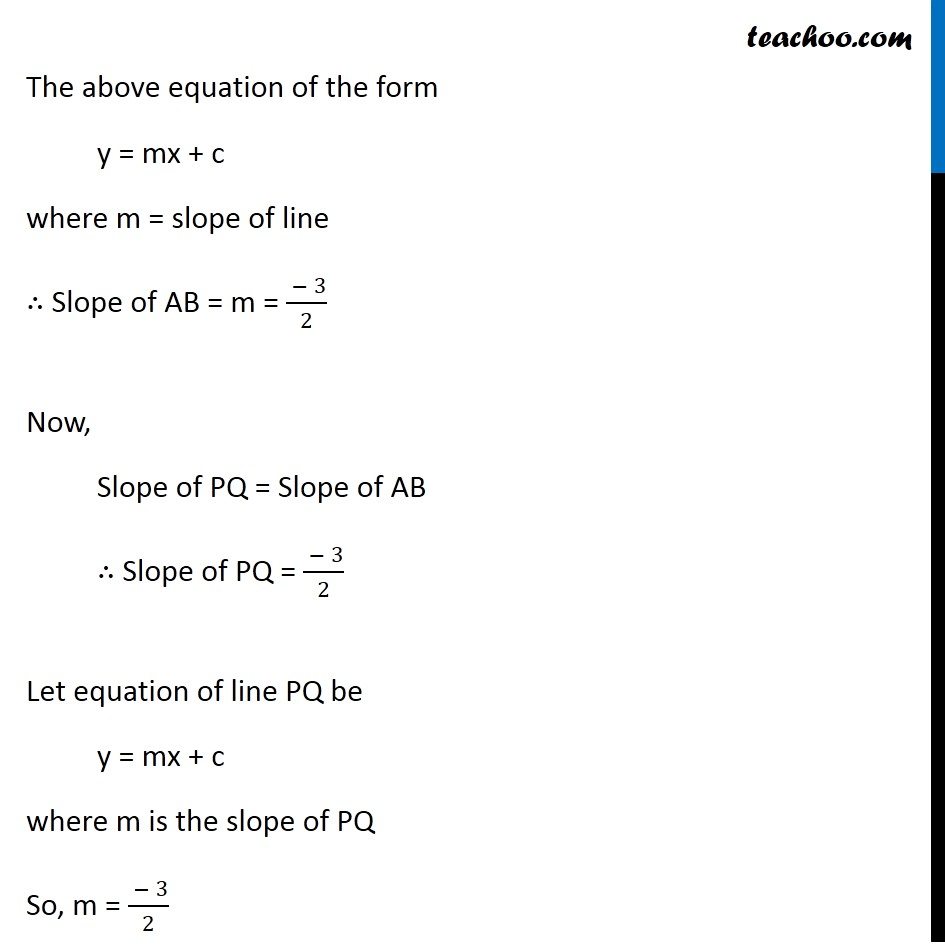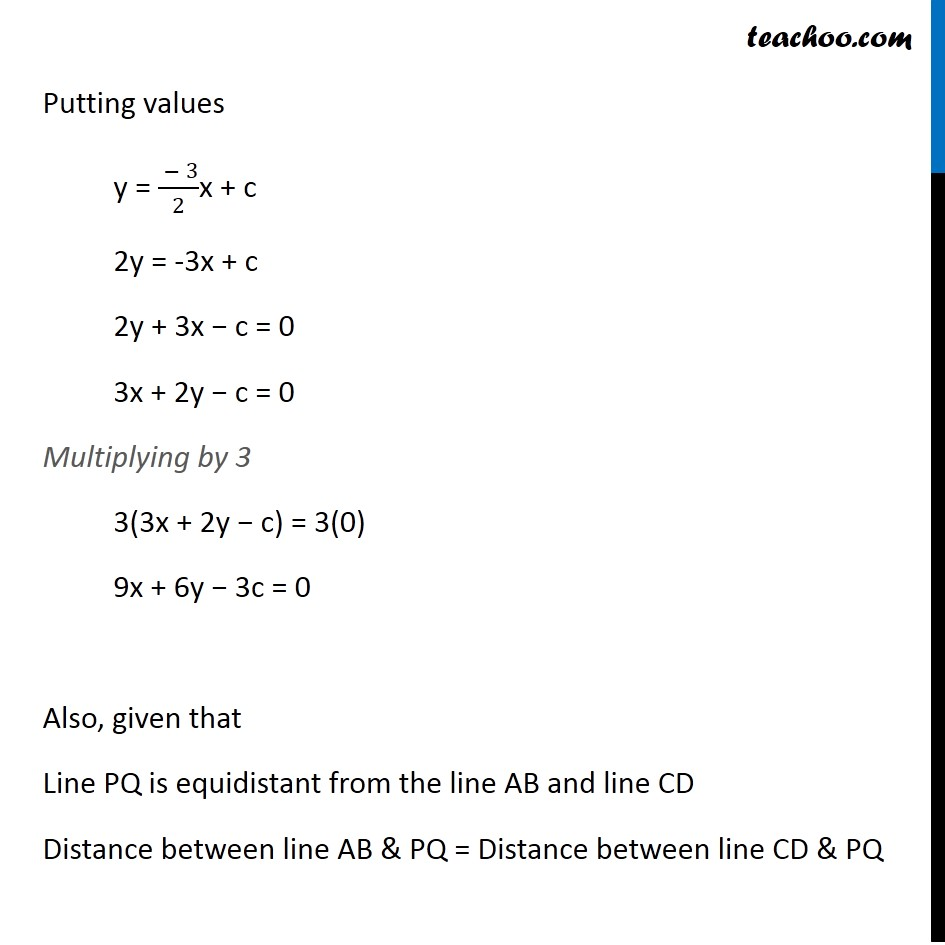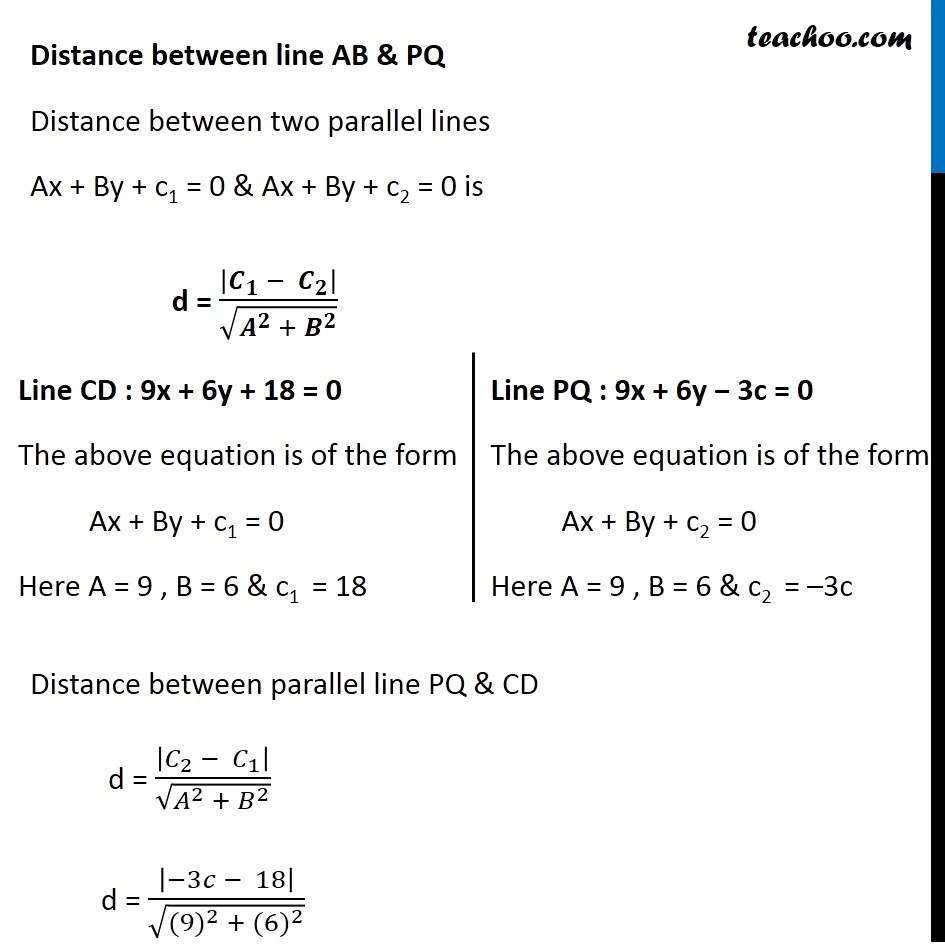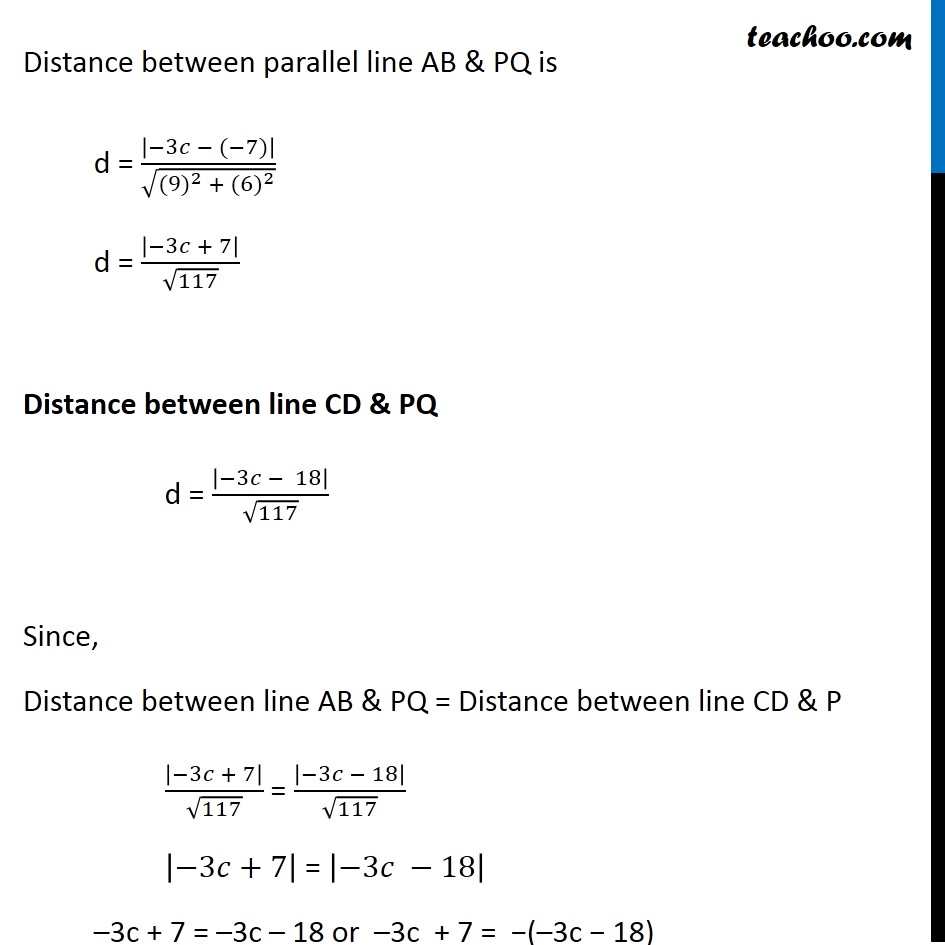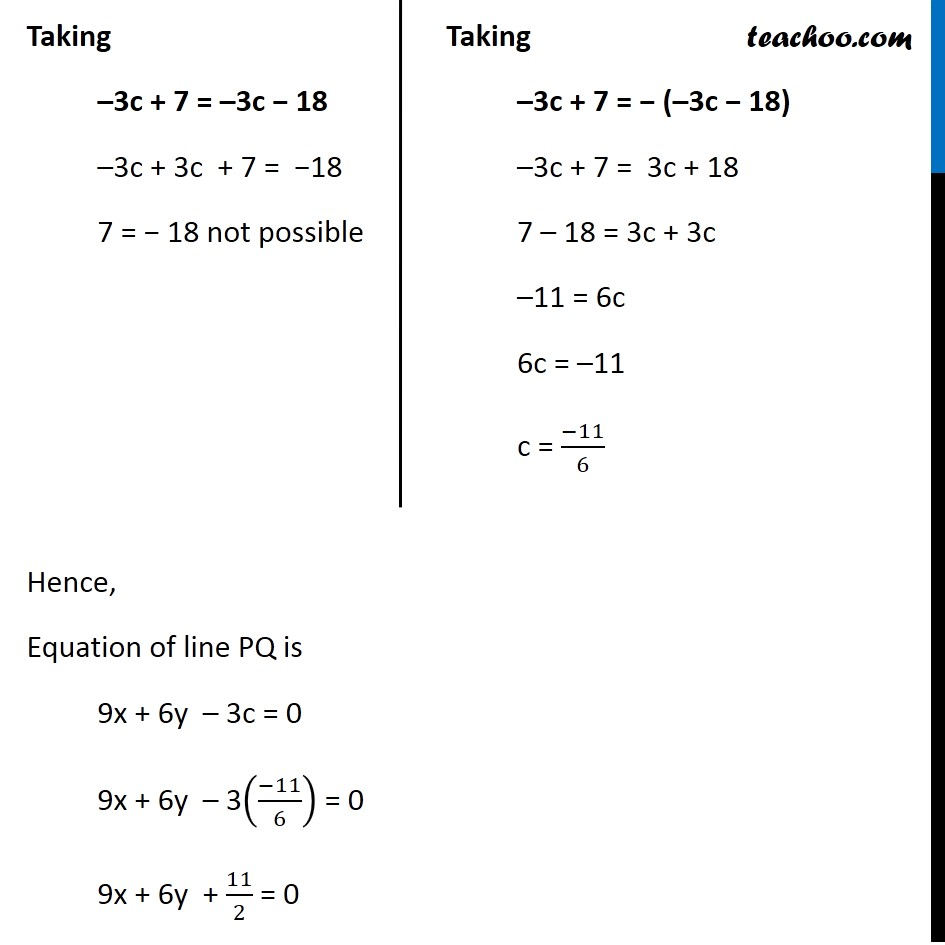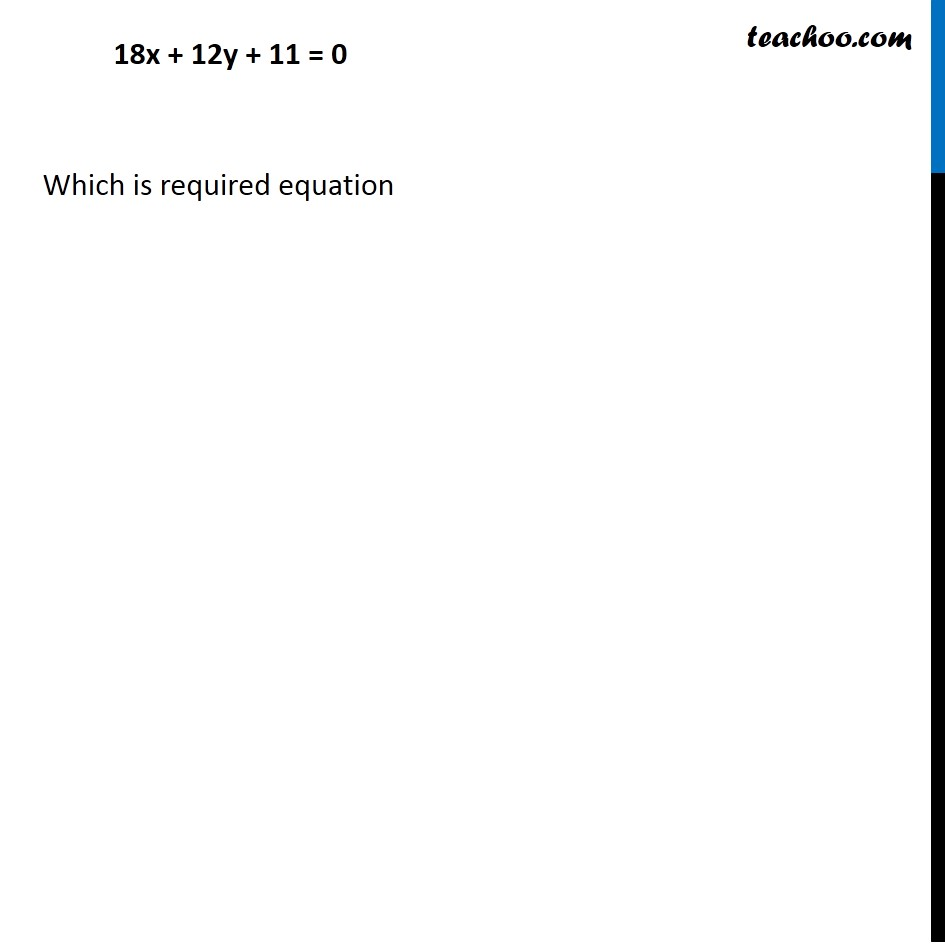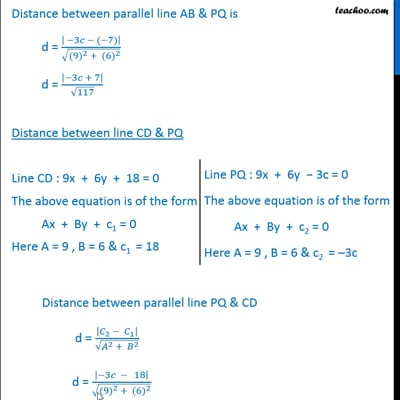This video is only available for Teachoo black users

Introducing your new favourite teacher - Teachoo Black, at only ₹83 per month

### Transcript

Misc 21 Find equation of the line which is equidistant from parallel lines 9x + 6y – 7 = 0 and 3x + 2y + 6 = 0. Let the given parallel lines be Line AB : 9x + 6y − 7 = 0 Line CD : 3x + 2y + 6 = 0 Multiplying equation of line CD by 3 3(3x + 2y + 6) = 3(0) 9x + 6y + 18 = 0 So equation of line CD is 9x + 6y + 18 = 0 Let the third line be PQ As PQ is equidistant from parallel lines AB & CD So, PQ parallel to the line AB & CD We need to find equation of line PQ Since Line PQ is parallel to the line AB & CD ∴ Slope of PQ = Slope of AB Finding slope of line AB 9x + 6y − 7 = 0 6y = 7 − 9x 6y = − 9x + 7 y = ( − 9𝑥 + 7)/6 y = (( − 9)/6)x + 7/9 y = (( − 3)/2)x + 7/9 The above equation of the form y = mx + c where m = slope of line ∴ Slope of AB = m = ( − 3)/2 Now, Slope of PQ = Slope of AB ∴ Slope of PQ = ( − 3)/2 Let equation of line PQ be y = mx + c where m is the slope of PQ So, m = ( − 3)/2 Putting values y = ( − 3)/2x + c 2y = -3x + c 2y + 3x − c = 0 3x + 2y − c = 0 Multiplying by 3 3(3x + 2y − c) = 3(0) 9x + 6y − 3c = 0 Also, given that Line PQ is equidistant from the line AB and line CD Distance between line AB & PQ = Distance between line CD & PQ Distance between line AB & PQ Distance between two parallel lines Ax + By + c1 = 0 & Ax + By + c2 = 0 is d = |𝑪_𝟏 − 𝑪_𝟐 |/√(𝑨^𝟐 + 𝑩^𝟐 ) Line CD : 9x + 6y + 18 = 0 The above equation is of the form Ax + By + c1 = 0 Here A = 9 , B = 6 & c1 = 18 Line PQ : 9x + 6y − 3c = 0 The above equation is of the form Ax + By + c2 = 0 Here A = 9 , B = 6 & c2 = –3c Distance between parallel line AB & PQ is d = |−3𝑐 − (−7)|/√(〖(9)〗^2 + 〖(6)〗^2 ) d = |−3𝑐 + 7|/√117 Distance between line CD & PQ d = |−3𝑐 − 18|/√117 Since, Distance between line AB & PQ = Distance between line CD & P |−3𝑐 + 7|/√117 = |−3𝑐 − 18|/√117 |−3𝑐+7| = |−3𝑐 −18| –3c + 7 = –3c – 18 or –3c + 7 = −(–3c − 18) Taking –3c + 7 = –3c − 18 –3c + 3c + 7 = −18 7 = − 18 not possible Taking –3c + 7 = − (–3c − 18) –3c + 7 = 3c + 18 7 – 18 = 3c + 3c –11 = 6c 6c = –11 c = (−11)/6 Hence, Equation of line PQ is 9x + 6y – 3c = 0 9x + 6y – 3((−11)/6) = 0 9x + 6y + 11/2 = 0 18x + 12y + 11 = 0 Which is required equation